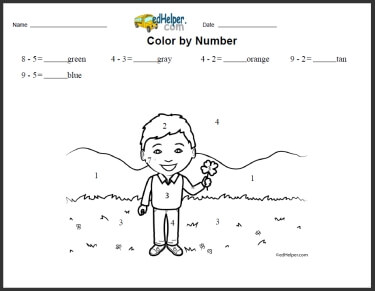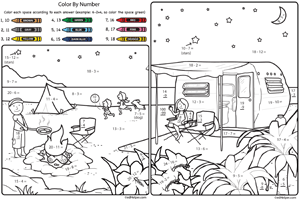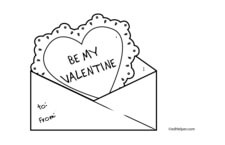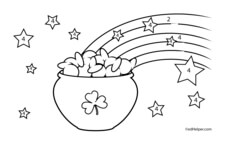Worksheets and No Prep Teaching Resources
Math Worksheets

# Free Color by Subtraction Worksheets

Students will "take away" a great time as they practice subtraction facts while doing amazing coloring sheets. These beautiful coloring sheets will effortlessly take students through subtraction facts and build a solid foundation for future math success.Color by Number SubtractionColor by Number: Subtraction - Camping

Math Lesson: Color by Numbers - February Color by Number BookSubtraction Coloring: a subtraction equation given for each color Addition and Subtraction Coloring: an addition or subtraction equation given for each color

Math Lesson: Color by Numbers - March Color by Number BookSubtraction Coloring: a subtraction equation given for each color Addition and Subtraction Coloring: an addition or subtraction equation given for each color

Math Lesson: Color by Numbers - August Color by Number BookSubtraction Coloring: a subtraction equation given for each color Addition and Subtraction Coloring: an addition or subtraction equation given for each color

Have a suggestion or would like to leave feedback?